Latest Banking jobs   »

# Quantitative Aptitude Quiz For LIC ADO Mains 2023- 20th April

Q1. Amit’s saving is 1000 Rs more than Vikas’s saving. They both save 10% of their respective income. Amit and Vikas both invested 30% and 20% of their respective income in a scheme which offers 10% per annum for 10 months and 12 months respectively. If total interest earned by both is Rs.2500, then find the income of Vikas?
(a) 60000
(b) 48000
(c) 35000
(d) 55000
(e) 50000

Q2. A Jar contain water and milk in the ratio of 2: 3. Some milk is added in the Jar whose amount is equal to 30% of water present in the Jar. After this some water is added whose amount is equal to 10% of quantity of milk present in Jar presently. What is the new ratio of water and milk in the Jar.
(a) 59 : 90
(b) 11 : 18
(c) 90 : 59
(d) 18 : 11
(e) 57 : 67

Q3. Bhavya have 10,000 Rs. He invested some amount in Scheme ‘A’ which offers 15% p.a. at SI and rest in Scheme ‘B’ which offers 20% p.a. at CI. Interest earned form scheme ‘A’ after 2 years is 780Rs more than interest earned form scheme ‘B’ after 2 years. Find the amount invested in Scheme ‘B’?
(a) 8000 Rs
(b) 7000 Rs
(c) 3000 Rs
(d) 2000 Rs
(e) 5000 Rs

Q4. A and B enter into a partnership with their respective capital of Rs 7500 and Rs 7800 for 2 years. B left the partnership ‘4 months’ before completion of 2 years and they together earn Rs 14000 profit at end of 2 years out of which 12.5% was donated and remaining was distributed according to their investment and time. Find profit share of B?
(a) Rs 4225.50
(b) Rs 6562.50
(c) Rs 7525.50
(d) Rs 5687.50
(e) None of these

Q5. A man invested Rs 3600 in ratio 7 : 6 : 5 in three schemes I, II and III which offers 10% p.a. at CI, 12% p.a. at SI and r% p.a. at CI respectively. If at the end of 2 years, he received total interest of Rs 1832, then Find the value of ‘r’?
(a) 30%
(b) 50%
(c) 25%
(d) 15%
(e) 20%

Q6. An amount is invested at Simple Interest for 2 years and after two years whole amount (Initial amount + Interest) is invested at Compound Interest for 2 more years. If rate of interest is 10% for both simple and compound interest and total interest earned after 4 years is 1130Rs. then, find the initial amount invested?
(a) 2500
(b) 3000
(c) 1500
(d) 1880
(e) 2340

Q7. Two jar A and B contain a mixture of two liquids, X and Y in the ratio of 2 : 3 and 4 : 5 respectively. If mixture from jar A and B are mixed in the ratio of 1 : 2 then, find the ratio of X and Y in final mixture.
(a) 70:51
(b) 58:77
(c) 61:77
(d) 77:58
(e) 62:61

Q8. Ramesh and Suresh enter into a partnership with ‘X’ Rs. and ‘X + 2000’ Rs. Suresh withdraw its investment after 8 months. If after one year their profit is equal then, find the value of ‘X’.
(a) 6000
(b) 2000
(c) 4000
(d) 8000
(e) 10000

Q9. A container whose capacity is 80 liter contains milk and water in the ratio 3 : 2. How much quantity of mixture should be replaced with milk so that in the final mixture, ratio of milk to water each 27 : 13?
(a) 16 litres
(b) 25 litres
(c) 28 litres
(d) 15 litres
(e) 32 litres

Q10. Arun invested Rs X in scheme I for three years at the rate of 12% p.a. at simple interest while Kush invested Rs (X + 4000) in scheme II for 2 years on C.I. at 20% p.a. rate. Find sum invested by Kush if total interest earned by them is Rs. 3360.
(a) 6000
(b) 4500
(c) 2000
(d) 3500
(e) 5200

Q11. A milkman made a mixture by mixing milk and water in the ratio 2 : 3 and sold 37.5% of the mixture. If he added 62.5 ℓ water in the remaining mixture, ratio of milk to water becomes 2 : 5, then Find the initial quantity of mixture?
(a) 325ℓ
(b) 400ℓ
(c) 250ℓ
(d) 150ℓ
(e) 450ℓ

Q12. Three partners A, B and C invested their amounts in ratio of 2 : 5 : 7. At the end of 6 months, ‘A’ added some more amount such that his investment become equals to half of sum of ‘B’ and ‘C’ initial investment. If at the end of the year, B’s share in profit is Rs 4250, then find the total profit?
(a) Rs 12500
(b) Rs 13600
(c) Rs 18400
(d) Rs 10500
(e)Rs 14500

Q13. Abhishek lent Satish Rs.12000 on C.I. at the rate of 20% per annum and at the end of first year Satish borrowed Rs. x more from Abhishek on C.I. at the same rate. If at the end of second year, Satish paid total amount of Rs.20400 to Abhishek then find how much extra amount Satish borrow at the end of first year?
(a) Rs.2400
(b) Rs.2000
(c) Rs.3600
(d) Rs.2600
(e) Rs.4000

Q14. Veer and Subham entered into partnership. Veer invested Rs.3x for first four month and Rs.5x for next six months and Subham invested Rs.1800 for 12 months. If Veer and Subham got profit share in the ratio of 7 : 9 then, find the value of ‘5x’ ?
(a) 2000 Rs.
(b) 1600 Rs.
(c) 2400 Rs.
(d) 3600 Rs.
(e) 4000 Rs.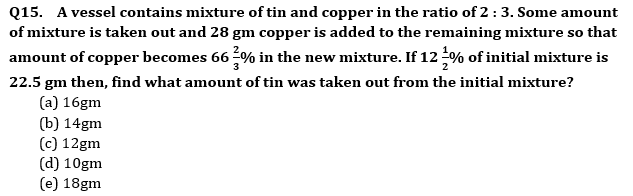Solutions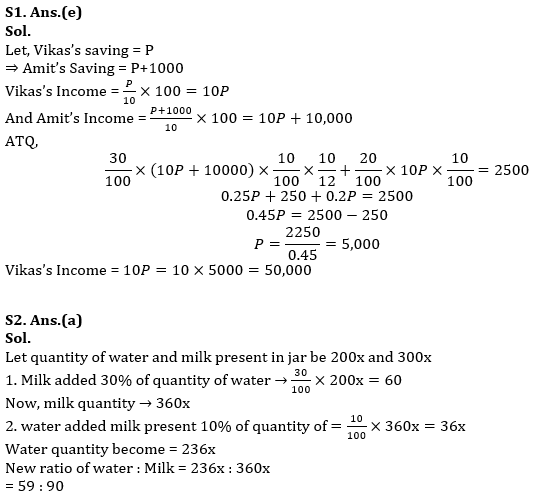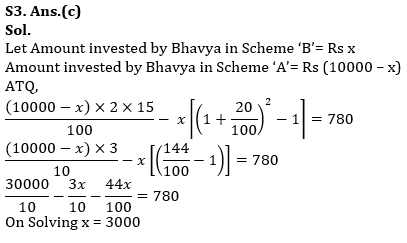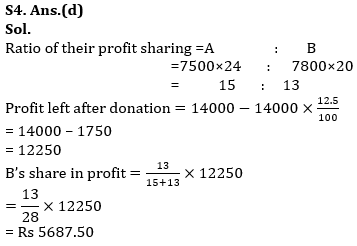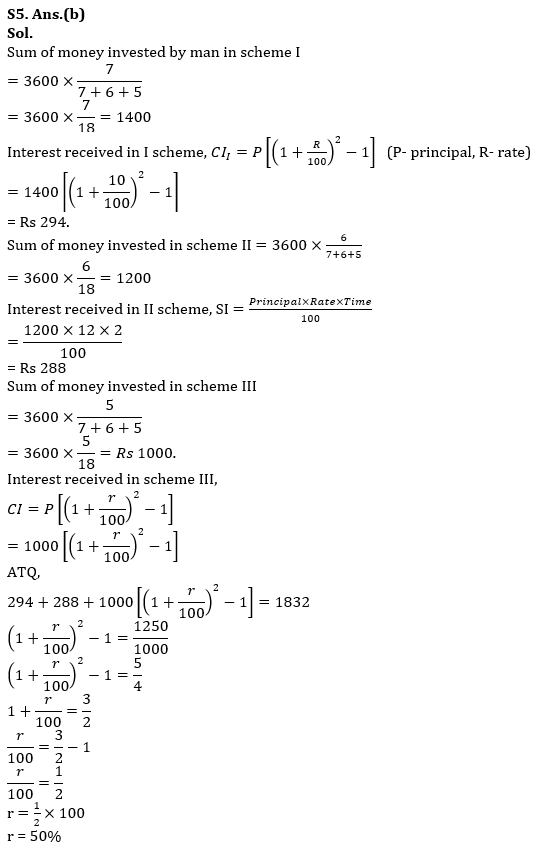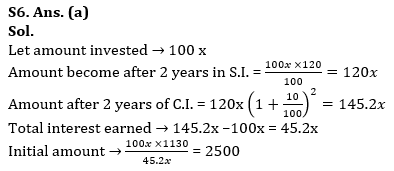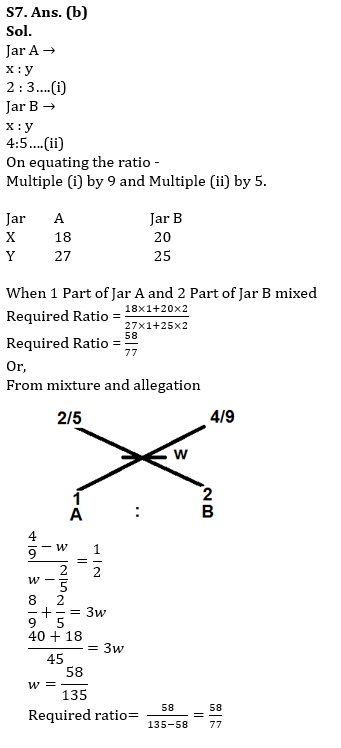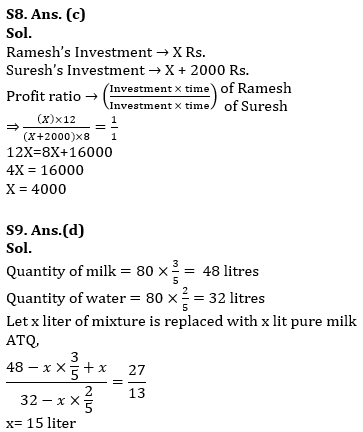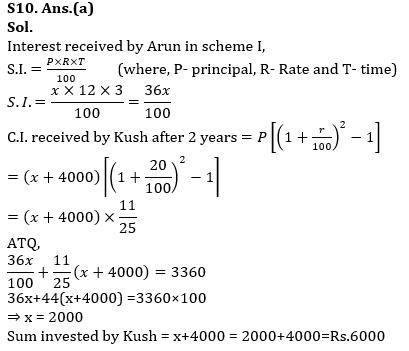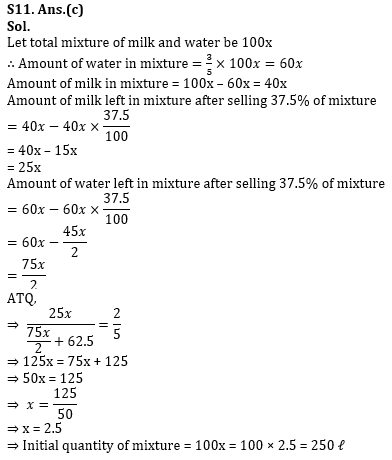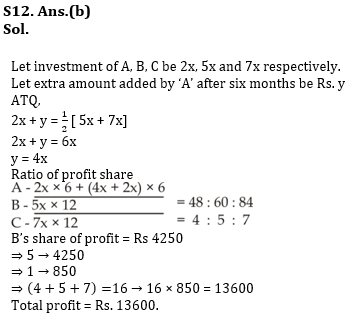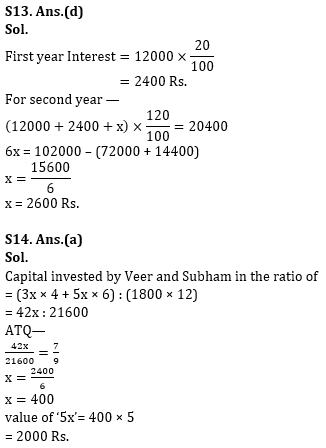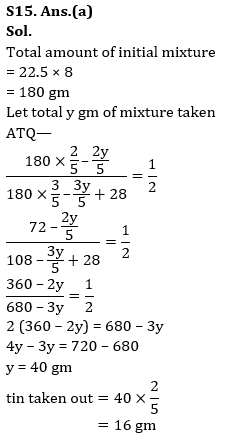## FAQs

### When is the LIC ADO Mains Exam scheduled?

The LIC ADO Mains exam is scheduled to be held on 23 April 2023

#### Congratulations!Union Budget 2023-24: Free PDF